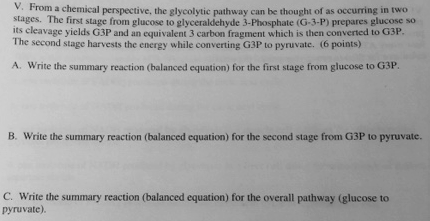# Problem: From a chemical perspective, the glycolytic pathway can be thought of as occurring in two stages. The first stage from glucose to glyceraldehyde 3-phosphate (G-3-P) prepares glucose so its cleavage yields G3P and an equivalent 3-carbon fragment which is then converted to G3P. The second stage harvests the energy while converting G3P to pyruvate.A. Write the summary reaction (balanced equation) for the first stage from glucose t G3P.B. Write the summary reaction (balanced equation) for the second stage from G3P to pyruvate.C. Writethe summary reaction (balanced equation) for the overall pathway (glucose to pyruvate)

###### FREE Expert Solution

The two phases of glycolysis are the preparatory phase and the payoff phase. The preparatory phase involves the steps that expend energy as investment as to convert glucose into products that can further pass into more exergonic reactions. The payoff phase are the series of reactions that result in the generation of ATP.###### Problem Details

From a chemical perspective, the glycolytic pathway can be thought of as occurring in two stages. The first stage from glucose to glyceraldehyde 3-phosphate (G-3-P) prepares glucose so its cleavage yields G3P and an equivalent 3-carbon fragment which is then converted to G3P. The second stage harvests the energy while converting G3P to pyruvate.

A. Write the summary reaction (balanced equation) for the first stage from glucose t G3P.

B. Write the summary reaction (balanced equation) for the second stage from G3P to pyruvate.

C. Writethe summary reaction (balanced equation) for the overall pathway (glucose to pyruvate)